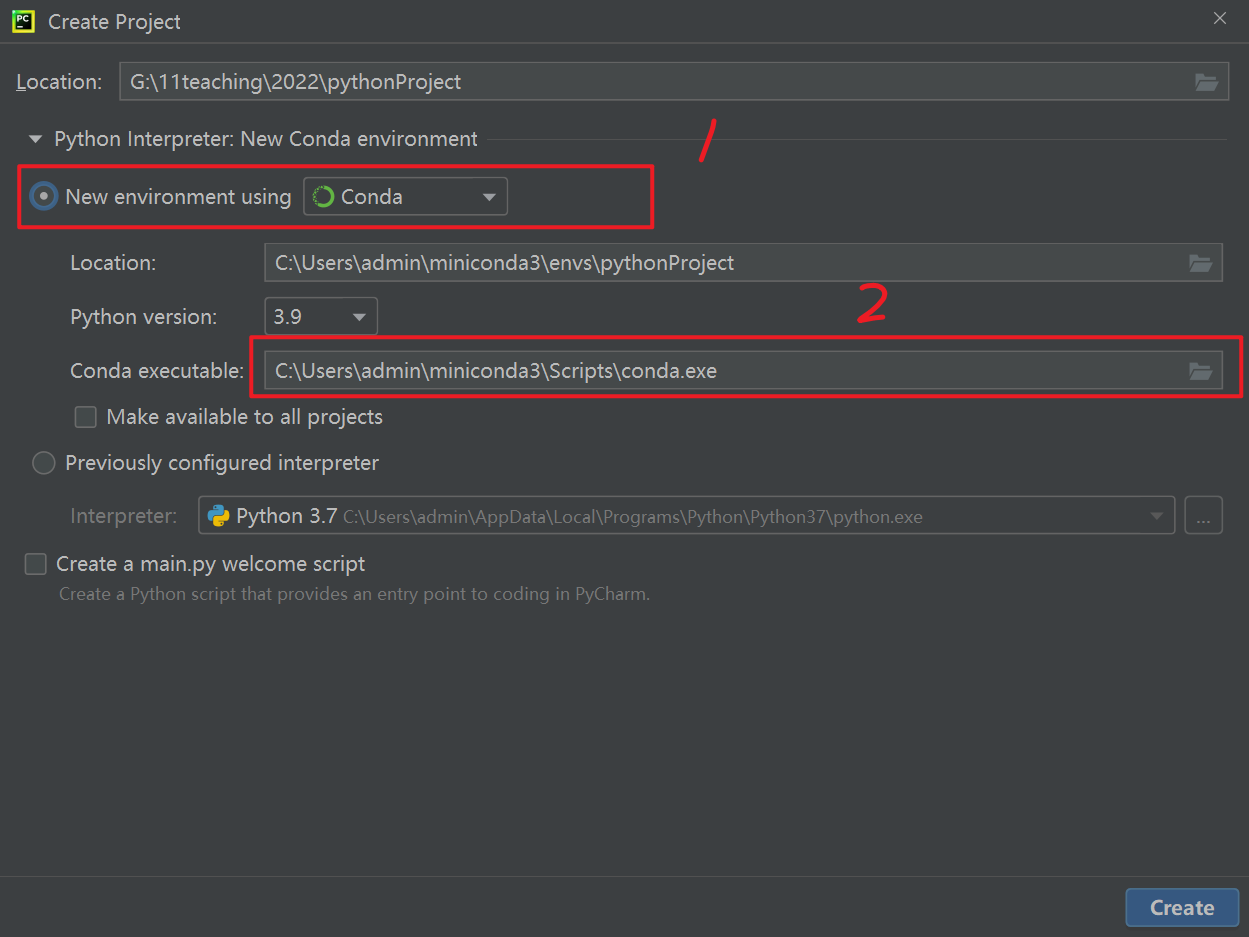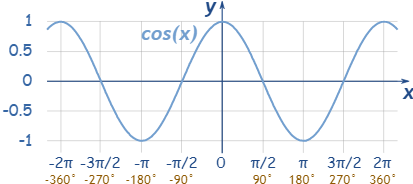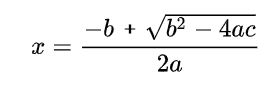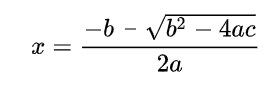• python一对一辅导
2021-01-30 15:49:29

Python编程辅导与R编程辅导

根据作业内容，协同完成编程任务、开发过程共享、问题解答。纯个人，中介勿扰。

服务流程：

前段。与学生一对一确认作业或问题细节，可以无障碍阅读全英文文档，判断出作业难度与完成时间点。

中段。进入正式开发过程，中间结果会及时发送给学生，与学生确认是否符合要求，并解释代码的作用

后段。交付代码，并安排一对一在线讲解代码，可以辅助学生对每一行代码写注释。如作业任务出现新要求，可以帮助学生修改代码。

个人简历

本人从2006年开始使用python，R开发以上，一线大厂经验，喜爱编程。

熟练如下技术：

Django

NLP

xgboost

linux

pandas

statistics computer

sklearn

pyDSTools

机器学习

数据分析

基本介绍：

英文读写流利。平时主要阅读英文文献

国内985, 211正规统招

个人业余产品如下：

辅导案例

2020年, McGill University, sklearn

MiniProject 1: Analyzing COVID-19 Search Trends and Hospitalization

COMP 551, Fall 2020, McGill University

2020年 University of Sydney, Crontab

This assignment involves developing a system to run programs at scheduled times. This is similar to the Unix and Linux "cron" system.

Epoch 6/10

1/1 [==============================] - 0s 976us/step - loss: 3.0003e-06 - accuracy: 1.0000

Epoch 7/10

1/1 [==============================] - 0s 1ms/step - loss: 9.7636e-07 - accuracy: 1.0000

Epoch 8/10

1/1 [==============================] - 0s 999us/step - loss: 1.4059e-06 - accuracy: 1.0000

Epoch 9/10

1/1 [==============================] - 0s 978us/step - loss: 7.4301e-07 - accuracy: 1.0000

Epoch 10/10

1/1 [==============================] - 0s 945us/step - loss: 6.7130e-07 - accuracy: 1.0000

Epoch 4

The Black Scholes model, also known as the Black-Scholes-Merton (BSM) model, is a mathematical model for pricing an options contract. In particular, the model estimates the variation over time of financial instruments. It assumes these instruments (such as stocks or futures) will have a lognormal distribution of prices. Using this assumption and factoring in other important variables, the equation derives the price of a call option.

DNA simulation, biological with R

Mini CGI Server

Generate all permutation of given string

House price predict

Eight Queue, backtrace

R timeseries analysis, ARIMA etc

Weibo sentiment analysis, python, nlp, 微博情感分析

三元事实组抽取

股票获利策略分析，timeseries analysis

联系我

Email: 164504252@qq.com

公众号: 行恒编程辅导更多相关内容
• 本博客为一对一辅导学生python代码的教案, 获得学生允许公开。 目前具体辅导内容为PyGeM 本文为PyGeM tutorial-1-ffd 的讲解与分析 Tutorial 1 shows how to apply the free-form deformation to mesh nodes. 0 ...

作者自我介绍：大爽歌, b站小UP主直播编程+红警三python1对1辅导老师
本博客为一对一辅导学生python代码的教案, 获得学生允许公开。
目前具体辅导内容为PyGeM

本文为PyGeM tutorial-1-ffd 的讲解与分析
Tutorial 1 shows how to apply the free-form deformation to mesh nodes.

### 0 准备工作

#### 准备软件

必须安装

1. python 3.8或以上版本
2. conda
conda 又分为 Anaconda 和 Miniconda
电脑性能足够好，推荐安装 Anaconda (这个是一个很大的软件)
性能一般或者不想安装太多太大的东西，可以尝试安装 Miniconda
Anaconda: https://www.anaconda.com/products/individual
Miniconda: https://docs.conda.io/en/latest/miniconda.html

#### 安装第三方库 pygem

PyGeM 不能直接使用pip来安装，需要按如下步骤来。 (准确来讲，是pip安装的不符合需求，版本太老)

推荐先使用Pycharm建立项目，
再在Pycharm的Terminal中安装。(这个也相当于命令行)
Pycharm 建立项目时 Python Interpreter建议选择如下1选Conda
2根据自己电脑上Conda的位置来选

先安装依赖库

1. 可以直接pip安装的有
numpy, scipy, matplotlib, sphinx, pytest
安装命令如下(命令行中运行)
pip install numpy
pip install scipy
pip install matplotlib
pip install sphinx
pip install pytest


安装不上可能需要使用镜像，
具体推荐阅读:
大爽Python入门教程 8-2 Python 库(Library)、包(Package)、模块(Module) 的第三方库部分。

1. 手动安装 pythonocc-core
要使用子模块submodule，则需要安装这个。
可以使用conda命令来安装
安装命令如下(命令行中运行)
conda install -c conda-forge pythonocc-core=7.4.0

1. 手动安装 PyGeM

安装命令如下(命令行中运行)

git clone https://github.com/mathLab/PyGeM


然后将控制台当前路径切换到这个项目的文件夹中去
如果在Pycharm的项目的Terminal 中运行的上面的代码的话，
这时Terminal切换路径的命令为

cd PyGeM


然后输入以下命令即可安装PyGeM

python setup.py install


到这里基本就安装好了

### 1 开头

#### 首段

原文与翻译

Free Form Deformation on a sphere
球体上的自由形式变形
In this tutorial we will show the typical workflow to perform a deformation on a generic geometry using the free-form deformation method implemented within PyGeM.
在本教程中，我们将展示使用 PyGeM 中实现的自由形式变形(FFD)方法对通用几何图形执行变形的典型工作流程。
A brief theoretical overview of the method is introduced in the pygem.ffd module,
pygem.ffd 模块中介绍了该方法的简要理论概述，
while in the README you can find several references that focus on FFD.
而在README中，您可以找到一些关注 FFD 的参考资料。

First of all, we import the required PyGeM class and we set matplotlib for 3D plots.
首先，我们导入所需的 PyGeM 类，并为 3D 绘图设置 matplotlib。
The version of PyGeM we are using in this tutorial is the 2.0.0.
我们在本教程中使用的 PyGeM 版本是 2.0.0。

#### 导入

原文代码

%matplotlib inline
import numpy as np
import mpl_toolkits.mplot3d
import matplotlib.pyplot as plt

import pygem
print(pygem.__version__)
from pygem import FFD


首行的%matplotlib inline是给 Ipython 编译器用的(可以在网页中做内嵌画图)，
常常是Jupyter或colab 的ipynb笔记本会用到。

一般.py文件不需要这个命令，注释或者删掉最好。

大爽Python入门教程 8-1 导入import

import math
print(math.pi)

from math import pi
print(pi)

import math as m
print(m.pi)


### 2 mesh_points

Then, the other ingredient is the original geometry we want to deform.
然后，另一个成分是我们想要变形的原始几何体。
In this simple tutorial we just span some points around a sphere and morph their coordinates using the FFD class.
在这个简单的教程中，我们只是跨越一个球体周围的一些点，并使用 FFD 类改变它们的坐标。

def mesh_points(num_pts = 2000):
indices = np.arange(0, num_pts, dtype=float) + 0.5

phi = np.arccos(1 - 2*indices/num_pts)
theta = np.pi * (1 + 5**0.5) * indices

return np.array([np.cos(theta) * np.sin(phi), np.sin(theta) * np.sin(phi), np.cos(phi)]).T

mesh = mesh_points()
plt.show()


#### phi

numpy.arange(start, stop, dtype = None)
Return evenly spaced values within a given interval.
在给定的间隔内返回均匀间隔的值。

>>> import numpy as np
>>> np.arange(0, 5, dtype=float)
array([0., 1., 2., 3., 4.])
np.arange(0, 5, dtype=float) + 0.5
array([0.5, 1.5, 2.5, 3.5, 4.5])
>>> np.arange(0, 2000, dtype=float) + 0.5
array([5.0000e-01, 1.5000e+00, 2.5000e+00, ..., 1.9975e+03, 1.9985e+03,
1.9995e+03])


关于e: 大爽Python入门教程 1-2 数与字符串

num_pts取默认值2000
indices = np.arange(0, 2000, dtype=float) + 0.5
得到了 0.5, 1.5, 2.5 一直到 1999.5 构成的数组

indices/num_pts 得到一个 1 分成 num_pts 份的数组
1 - 2* indices/num_pts得到一个[-1, 1]之间分成num_pts 份的数组

np.arccos(1 - 2*indices/num_pts)得到的是 [ 0 , π ] [0, \pi] 之间的的数构成的数组（此时不是均等分隔的了）
是如图y轴上均等分隔的点对应到曲线上的点的横坐标。phi 对应球坐标系的 ϕ \phi
具体见后面的椭球公式

#### theta

np.pi * (1 + 5**0.5) * indices
这个就是 π ∗ ( 1 + 5 ) \pi * (1+ \sqrt 5)

这个是用于去得到坐标得，
采用得大概是黄金螺旋线法
详细原理我也不太明白，
这里就不深究了，能用就行。

感兴趣可以去看下这个:
evenly-distributing-n-points-on-a-sphere

#### return

np.array([np.cos(theta) * np.sin(phi), np.sin(theta) * np.sin(phi), np.cos(phi)])
会得到一个3行num_pts列的

[[ 0.05111927 -0.21801231  0.29980505 -0.19082906  ......  -0.17335079  0.14097948]
[-0.13147935  0.10756687  0.08727502 -0.31414053  ......  0.17043915 -0.0049786 ]
[ 0.99        0.97        0.95        0.93        ......  -0.97       -0.99      ]]


加个 .T得到 num_pts行3列的

[[ 0.05111927 -0.13147935  0.99      ]
[-0.21801231  0.10756687  0.97      ]
[ 0.29980505  0.08727502  0.95      ]
[-0.19082906 -0.31414053  0.93      ]
......
[-0.17335079  0.17043915 -0.97      ]
[ 0.14097948 -0.0049786  -0.99      ]]


#### 椭球公式

椭球

球坐标系，其中 φ \varphi 是天顶角， θ \theta 是方位角，则椭球可以表示为以下的参数形式：
x = a sin ⁡ φ cos ⁡ θ ; y = b sin ⁡ φ sin ⁡ θ ; z = c cos ⁡ φ ; 0 ≤ φ ≤ 18 0 ∘ ; 0 ≤ θ ≤ 36 0 ∘ ; x = a \sin \varphi \cos \theta; \\ y = b \sin \varphi \sin \theta;\\ z = c \cos \varphi;\\ 0 \leq \varphi \leq 180 ^\circ ; 0 \leq \theta \leq 360 ^\circ ; \\### 3 第一张图

原文代码

import numpy as np
import matplotlib.pyplot as plt

def mesh_points(num_pts = 2000):
indices = np.arange(0, num_pts, dtype=float) + 0.5

phi = np.arccos(1 - 2*indices/num_pts)
theta = np.pi * (1 + 5**0.5) * indices

return np.array([np.cos(theta) * np.sin(phi), np.sin(theta) * np.sin(phi), np.cos(phi)]).T

mesh = mesh_points()
plt.show()


运行效果如图这里来详细看下绘图部分代码

plt.figure(figsize=(8,8)).add_subplot(111, projection='3d').scatter(*mesh.T);
plt.show()


#### figure

plt.figure(figsize=(8,8))
Create a new figure, or activate an existing figure.
figsize 指定 Width, height in inches.

figure可以认为是绘图的窗口。

示例代码

import matplotlib.pyplot as plt
fig = plt.figure(figsize=(8,8))
plt.show()


运行效果如下一个空的绘图窗口

#### subplot

该方法用于在窗口figure里面添加或规划轴线axes

plt.subplot(*args, **kwargs)
Add an Axes to the current figure or retrieve an existing Axes.
将轴添加到当前图窗或检索现有轴。
This is a wrapper of Figure.add_subplot which provides additional behavior when working with the implicit API (see the notes section).
这是 Figure.add_subplot 的包装器，它在使用隐式 API 时提供额外的行为（请参阅注释部分）。

Call signatures:

subplot(nrows, ncols, index, **kwargs)  # 位置
subplot(pos, **kwargs)  # 位置
subplot(**kwargs)
subplot(ax)


示例: tutorial_101d.py如下

import matplotlib.pyplot as plt

# 按 三行x二列 的布局分割窗口，
# 得到总共六块区域
# 在第1个区域，放下第1个轴线 ax1
# plt.subplot(321)
# 下面的和上面的效果相同，下面的写法更正式
ax1 = plt.subplot(3, 2, 1)

# 在第2个区域，放下第2个轴线 ax2
# 该轴线无边框
ax2 = plt.subplot(322, frameon=False)

# 在第3个区域，放下第3个轴线 ax3
# 该轴线为极坐标轴线
ax3 = plt.subplot(323, projection='polar')

# 在第4个区域，放下第4个轴线 ax4
# 该轴线背景色为红色
ax4 = plt.subplot(325, facecolor='red')

plt.show()


运行效果如下图#### 3d

fig.add_subplot(111, projection='3d')

• 111:在窗口中按一行x一列布局，在第一块区域放置轴线，
• projection='3d': 一个3d图轴线

实例代码

import matplotlib.pyplot as plt

fig = plt.figure(figsize=(8,8))

plt.show()


运行效果如下图3d图的三轴具体如下#### scatter

scatter(xs, ys, zs)
绘制散点图
根据指定的x, y, z 坐标

示例tutorial_101f.py如下

import matplotlib.pyplot as plt

fig = plt.figure(figsize=(8,8))

xs = [i for i in range(10)]
ys = [0 for i in range(10)]
zs = [0 for i in range(10)]

ax.scatter(xs, ys, zs)

plt.show()


运行效果如下#### 绘图代码拆解

plt.figure(figsize=(8,8)).add_subplot(111, projection='3d').scatter(*mesh.T);


这一句长代码，拆解成一步一步的话，
等价于如下代码

fig = plt.figure(figsize=(8,8))
xs, ys, zs = mesh.T
ax.scatter(xs, ys, zs)


还可以写成如下形式。

plt.figure(figsize=(8,8))
xs, ys, zs = mesh.T
plt.scatter(xs, ys, zs)

展开全文pycharm 开发语言
• Python一对一题目辅导「PTA 题目讲解·难度系数：基础」 题目范围 作业 7: 6-2编写函数计算一个或不特定多个数的乘积(高教社，《Python编程基础及应用》习题8-6)(4分) 1、函数接口定义： def caculate(*t) 打 * 号的...

点击此链接获得完整文章阅读：

Python一对一题目辅导「PTA 题目讲解·难度系数：基础」

# 作业 7:

## 6-2 编写函数计算一个或不特定多个数的乘积(高教社，《Python编程基础及应用》习题8-6) (4分)

### 1、函数接口定义：

def caculate(*t)

打 * 号的参数 t 接受多个参数成员，参数成员预期为整数或浮点数类型。

#### *args 的用法

*args**kwargs 主要用于函数定义。 你可以将不定数量的参数传递给一个函数。这里的不定的意思是：预先并不知道，函数使用者会传递多少个参数给你，所以在这个场景下使用这两个关键字。*args 是用来发送一个非键值对的可变数量的参数列表给一个函数。
这里有个例子帮你理解这个概念：

def test_var_args(*args):
print(args)
print(type(args))

test_var_args('yasoob', 'python', 'eggs', 'test')

# 输出
('yasoob', 'python', 'eggs', 'test')
<class 'tuple'>

我们可以发现，拿到的数据的数据类型是元组(tuple)

def test_var_args(f_arg, *argv):
print("first normal arg:", f_arg)
for arg in argv:
print("another arg through *argv:", arg)

test_var_args('yasoob', 'python', 'eggs', 'test')

# 输出
first normal arg: yasoob
another arg through *argv: python
another arg through *argv: eggs
another arg through *argv: test

*args：可以自行换成别的名称，不过我们约定俗成写成：args

我希望这解决了你所有的困惑. 那接下来让我们谈谈 **kwargs

#### **kwargs 的用法

**kwargs 允许你将不定长度的键值对, 作为参数传递给一个函数。 如果你想要在一个函数里处理带名字的参数, 你应该使用**kwargs

def greet_me(**kwargs):
print(kwargs)
print(type(kwargs))
for key, value in kwargs.items():
print("{0} == {1}".format(key, value))
# print("{1} == {0}".format(key, value)) # 第二个主要用来让同学们了解 format

greet_me(name="yasoob", gzh='aiyuechuang', the_public='AI悦创')

# 输出
{'name': 'yasoob', 'gzh': 'aiyuechuang', 'the_public': 'AI悦创'}
<class 'dict'>
name == yasoob
gzh == aiyuechuang
the_public == AI悦创

现在你可以看出我们怎样在一个函数里, 处理了一个键值对参数了。
这就是 **kwargs 的基础, 而且你可以看出它有多么管用。 接下来让我们谈谈，你怎样使用 *args**kwargs 来调用一个参数为列表或者字典的函数。

#### 使用 *args 和 **kwargs 来调用函数

那现在我们将看到怎样使用*args**kwargs 来调用一个函数。 假设，你有这样一个小函数：

def test_args_kwargs(arg1, arg2, arg3):
print("arg1:", arg1)
print("arg2:", arg2)
print("arg3:", arg3)

可以联想如果参数很多的话，这样就代码就不是非常的简洁。你可以使用 *args 或 **kwargs 来给这个小函数传递参数。 下面是怎样做：

# 首先使用 *args
>>> args = ("two", 3, 5)
>>> test_args_kwargs(*args)
arg1: two
arg2: 3
arg3: 5

# 现在使用 **kwargs:
>>> kwargs = {"arg3": 3, "arg2": "two", "arg1": 5}
>>> test_args_kwargs(**kwargs)
arg1: 5
arg2: two
arg3: 3

#### 标准参数与 *args、**kwargs 在使用时的顺序

那么如果你想在函数里同时使用所有这三种参数， 顺序是这样的：

some_func(fargs, *args, **kwargs)

#### 什么时候使用它们？

这还真的要看你的需求而定。
最常见的用例是在写函数装饰器的时候。
此外它也可以用来做猴子补丁(monkey patching)。猴子补丁的意思是在程序运行时(runtime)修改某些代码。 打个比方，你有一个类，里面有个叫 get_info 的函数会调用一个 API 并返回相应的数据。如果我们想测试它，可以把 API 调用替换成一些测试数据。例如：

import someclass

def get_info(self, *args):
return "Test data"

someclass.get_info = get_info

我敢肯定你也可以想象到一些其他的用例。

### 2、裁判测试程序样例：

s = input().split()            #接受输入字符串并按空格分拆，存入列表，列表成员为字符串类型
t = [float(x) for x in s]    #使用列表推导将列表s中的成员全部转换成浮点数，存入另一个列表t
print("%.4f" % caculate(*t))

### 3、输入样例：

3 2 1

### 4、输出样例：

6.0000

### 5、Coder

def caculate(*t):
product = 1
for value in t:
product *= value
return product

## 6-3 打印指定范围内的全部回文素数(高教社，《Python编程基础及应用》习题8-7) (6分)

知识点：
回文素数是指一个数既是素数又是回文数，例如 131 既是素数又是回文数。
请实现下述两个函数，帮助测试程序完成如下功能：从键盘输入正整数 N， 打印从 1 ~ N (包含 N )的全部回文素数，一行一个。

回文素数是指，对一个整数 n(n≥11)从左向右从右向左读：其结果值相同且是素数「质数」，即称 n 为回文素数。「1、实现方法」

除了 11，偶数位的数不存在回文质数。4位，6位，8位……数不存在回文质数。因为四位及四位以上的偶数位的回文数都可以被 11 整除，故不存在偶数位的回文质数。「2、判断输入数的位数」
最初几个回文素数：11，101，131，151，181，191，313，353，373，383，727，757，787，797，919，929……两位回文素数1个，三位回文素数15个，五位回文素数93个，七位回文素数668个，九位回文素数 5172个。

### 1、函数接口定义：

def isPrime(num):

def reverseNumber(num):

isPrime() 用于判断整数 num 是否是素数，是返回 True , 否则返回 False。
reverseNumber() 用于返回整数 num 的反向数，321 的反向数为 123， 320 的反向数为 23。

### 2、裁判测试程序样例：

N = int(input()) # 获取数字并转为整数，并且这个 N 是一个范围
for n in range(1, N+1): # range 是左闭右开，不会提取到结尾的那个数所以需要 + 1，然后以这个范围做循环。
if isPrime(n) and reverseNumber(n) == n:
print(n)

### 3、输入样例：

400

### 4、输出样例：

2
3
5
7
11
101
131
151
181
191
313
353
373
383

### 5、Coder

#### 1、质数的判断方法：

一个大于1的自然数，除了1和它本身外，不能被其他自然数(质数)整除(2, 3, 5, 7等)，换句话说就是该数除了1和它本身以外不再有其他的因数。方法一：

# 用户输入数字
num = int(input("请输入一个数字: "))

# 质数大于 1
if num > 1:
# 查看因子
for i in range(2, num):
if (num % i) == 0: # 肯定是除于比 num 小的数来检测是否能被整除
print(num,"不是质数")
print(i, "乘于", num//i, "是", num) # 如果能被整除那直接用 // 也是可以的。
break
else:
print(num, "是质数")

# 如果输入的数字小于或等于 1，不是质数
else:
print(num, "不是质数")

方法二：

from math import sqrt
def is_prime(n):
if n == 1:
return False
for i in range(2, int(sqrt(n))+1):
if n % i == 0:
return False
return True

**方法三：

def isPrime(num):
if num == 1:
return 0
elif num == 2:
return 2
else :
from math import sqrt
active = True
for i in range(2, int(sqrt(num))+1):
if num % i == 0:
active = False
if active:
return num
else:
return 0

#### 2、整数反转

解题思路：
首先我们想一下，怎么去反转一个整数？——用栈？
或者把整数变成字符串，再去反转这个字符串？
这两种方式是可以，但并不好。实际上我们只要能拿到这个整数的末尾数字就可以了。
以 12345 为例，先拿到 5，再拿到 4，之后是 3，2，1，我们按这样的顺序就可以反向拼接处一个数字了，也就能达到 反转 的效果。
怎么拿末尾数字呢？好办，用取模运算就可以了。「取余运算即可」

1、将 12345 % 10 得到 5，之后将12345 / 10
2、将1234 % 10 得到4，再将1234 / 10
3、将123 % 10 得到3，再将123 / 10
4、将12 % 10 得到2，再将12 / 10
5、将1 % 10 得到1，再将1 / 10

这么看起来，一个循环就搞定了，循环的判断条件是 x>0
但这样不对，因为忽略了 负数
循环的判断条件应该是 while(x!=0)，无论正数还是负数，按照上面不断的 /10 这样的操作，最后都会变成0，所以判断终止条件就是 !=0
有了取模和除法操作，对于像 12300 这样的数字，也可以完美的解决掉了。

看起来这道题就这么解决了：

def reverseNumber(num):
res = 0
while (num != 0):
tmp = num % 10
res = res * 10 + tmp
num = int(num / 10)
return res

if __name__ == '__main__':
print(reverseNumber(3456))

## 6-4 整数数位和(高教社，《Python编程基础及应用》习题8-3) (4分)

编写一个函数，该函数接受一个正整数作为参数，返回该整数的各位数字之和。

### 1、函数接口定义：

def digitSum(v)

v 为输入整数(正整数)； 函数返回一个整数，其值为 v 的各位数字之和。

### 2、裁判测试程序样例：

a = int(input())
print(digitSum(a))

### 3、输入样例：

291

### 4、输出样例：

12

### 5、Coder

def digitSum(a):
num_list = list(str(a))
tmp = 0
for i in num_list:
# tmp = tmp + int(i)
tmp += int(i)
return tmp
def digitSum(v):
num_list = list(str(v))
a = 0
for i in range(0,len(num_list)):
a += int(num_list[i])
return a

题目已经给定了这个数字，如果是在实际的开发当中，是需要对输入的数字是不是纯数字进行判断的。

## 6-5 编写函数计算f(i) = 1/2 + 2/3 + 3/4 + ... + i/(i+1) (高教社，《Python编程基础及应用》习题8-4) (4分)

编写函数计算f(i) = 1/2 + 2/3 + 3/4 + ... + i/(i+1) 。

### 1、函数接口定义：

def f(i):

i 为正整数，返回结果浮点数。

### 2、裁判测试程序样例：

v=int(input())
print("%.4f" % f(v))

### 3、输入样例：

5

### 4、输出样例：

3.5500

### 5、Coder

def f(i) :
result = 0
for x in range(i+1):
current_num = x / (x + 1)
result += current_num
return result

# 作业 8:

## 6-1 定义并实现身体质量指数类(高教社，《Python编程基础及应用》习题9-3) (4分)

按图施工，设计下述 BMI 「Body Mass Index」类，用于计算身体质量指数。该指数等于体重(kg) 除以身高 (米) 的平方。

相应的判定标准如下图所示。

### 1、BMI 类接口定义：

class BMI:
...

其中，name 为姓名，age 为年龄，height 为身高，单位为米，weight 为体重，单位为 kg。
getBMI() 函数返应计算所得的身体质量指数；
getStatus() 返回评价结果，其值应为超轻/标准/超重/肥胖之一。

### 2、裁判测试程序样例：

>>>x = 7
>>> eval( '3 * x' )
21
>>> eval('pow(2,2)')
4
>>> eval('2 + 2')
4
>>> n=81
>>> eval("n + 4")
85
sName = input()  #输入姓名
iAge = int(input()) #输入年龄
fHeight = eval(input())  #输入身高，预期为浮点数，单位米
fWeight = eval(input())  #输入体重，预期为浮点数，单位千克
bmi=BMI(sName,iAge,fHeight,fWeight) #实例化BMI类
print("Name:",bmi.name)
print("Age:",bmi.age)
print("BMI = %.3f" % bmi.getBMI())
print("Result =",bmi.getStatus())

### 3、输入样例：

Alex
27
1.75
68

### 4、输出样例：

Name: Alex
Age: 27
BMI = 22.204
Result = 标准

### 5、Coder

class BMI:
def __init__(self,sName,iAge,fHeight,fWeight):
self.name=sName
self.age=iAge
self.height=fHeight
self.weight=fWeight
def getStatus(self):
m=fWeight/(fHeight**2)
if m<18:
return ("超轻")
elif m>=18 and m<25:
return ("标准")
elif m>=25 and m<27:
return ("超重")
else:
return ("肥胖")
def getBMI(self):
n=fWeight/(fHeight**2)
return n

## 6-2 定义并实现 Book 类及其__del__函数(高教社，《Python编程基础及应用》习题9-4) (3分)

图书馆里有很多的书， 请定义一个名为 Book 的类。
该类的属性包括书名(字符串)，书号(字符串)，单价(浮点数)；
该类的构造函数接受书名，书号及单价三个参数并参成对象初始化；
该类的 del() 函数则向终端打印如下信息：Book destroyed-书名,书号,单价
注意：单价保留两位小数。

### 1、类接口定义：

class Book:
...

### 2、裁判测试程序样例：

sName = input()  #输入书名
sNo = input() #输入书号
fPrice = float(input())   #输入单价
b = Book(sName,sNo,fPrice)
b = None   #触发b对象的__del__方法的执行

### 3、输入样例：

Python编程基础及应用
888-999
43.678

### 4、输出样例：

Book destroyed-Python编程基础及应用,888-999,43.68

### 5、Coder

class Book:
def __init__(self,name,No,price):
self.sName=name
self.sNo=No
self.fPrice=price
def name(self):
return self.sName
def number(self):
return self.sNo
def price(self):
return self.fPrice
def __del__(self):
print("Book destroyed-%s,%s,%.2f" %(self.sName,self.sNo,self.fPrice))

创建对象后，python 解释器默认调用init()方法。当删除一个对象时，python解释器也会默认调用一个方法，这个方法为del()方法。在python中，对于开发者来说很少会直接销毁对象(如果需要，应该使用del关键字销毁)。Python的内存管理机制能够很好的胜任这份工作。也就是说,不管是手动调用del还是由python自动回收都会触发del方法执行：

import time

class Animal(object):
# 初始化方法
# 创建完对象后会自动被调用
def __init__(self, name):
print('__init__方法被调用')
self.__name = name

# 析构方法
# 当对象被删除时，会自动被调用
def __del__(self):
print("__del__方法被调用")
print("%s对象马上被干掉了..." % self.__name)

# 创建对象
dog = Animal("哈皮狗")

# 删除对象
del dog
cat = Animal("波斯猫")
cat2 = cat
cat3 = cat
print("---马上 删除cat对象")
del cat
print("---马上 删除cat2对象")
del cat2
print("---马上 删除cat3对象")
del cat3
print("程序2秒钟后结束")
time.sleep(2)

## 6-3 设计一元二次方程求解类(高教社，《Python编程基础及应用》习题9-4) (4分)

设计一个类 Root 来计算 ax+bx+c=0 的根。
该类包括：a、b、c 共 3 个属性表示方程的 3 个系数，getDiscriminant() 方法返回 b-4ac
getRoot1() 和 getRoot2() 返回方程的两个根。
其中，getRoot1()返回的根对应：getRoot2()返回的根对应：### 1、类接口定义：

class Root:
def __init__(self,a,b,c):
...

### 2、裁判测试程序样例：

a=float(input()) #请输入二次项系数
b=float(input()) #请输入一次项系数
c=float(input()) #请输入常数项系数

root=Root(a,b,c)
if root.getDiscriminant()>0:
print("{:.2f}".format(root.getRoot1()))
print("{:.2f}".format(root.getRoot2()))
elif root.getDiscriminant()==0:
print("{:.2f}".format(root.getRoot1()))
else:
print("No Root!")

### 3、输入样例：

2.1
10.2
3.0

### 4、输出样例：

-0.31
-4.54

### 5、Coder

from math import sqrt

class Root:
def __init__(self, a, b, c):
self.two = a
self.one = b
self.cs = c

def getDiscriminant(self):
return b ** 2 - 4 * a * c

def getRoot1(self):
m = b ** 2 - 4 * a * c
n = b * (-1) + sqrt(m)
return n / (2 * a)

def getRoot2(self):
m = b ** 2 - 4 * a * c
n = b * (-1) - sqrt(m)
return n / (2 * a)

## 6-4 设计一个股票类(高教社，《Python编程基础及应用》习题9-6) (4分)

设计一个名为 Stock 的类来表示一个公司的股票，包括以下内容：
1)股票代码、股票名称、前一天股票价格、当天股票价格4个私有属性；
2)构造方法，需初始化代码、名称、前一天价格和当天价格等属性；
3)返回股票名字的 get 方法;
4)返回股票代码的 get 方法;
5)获取和设置股票前一天价格的get和set方法;
6)获取和设置股票当前价格的get和set方法;
7)名为getChangePercent()方法，返回前日收市价至当前价格的变化百分比；
8). 包括文档字符串，其内容为:"Stock Information Class"
说明：各方法的名称及参数要求请参见测试程序。

### 1、类接口定义：

class Stock:
...

### 2、裁判测试程序样例：

# 股票代码、股票名称、前一天股票价格、当天股票价格 4 个私有属性；
sCode = input() #输入股票代码
sName = input() #输入股票名称
priceYesterday = float(input())  #输入前一天股票价格
priceToday = float(input())  #输入今日股票价格

# 类：Stock
s = Stock(sCode,sName,priceYesterday,priceToday) # 初始化代码

print("代码:", s.getCode())
print("名称:", s.getName())
print("昨日价格:%.2f\n今天价格:%.2f" % (s.getPriceYesterday(),s.getPriceToday()))
s.setPriceYesterday(50.25)
print("修正昨日价格为:%.2f" % 50.25)
print("价格变化百分比:%.2f%%" % (s.getChangePercent()*100))
print(Stock.__doc__)

### 3、输入样例：

601318
中国平安
63.21
64.39

### 4、输出样例：

代码: 601318
名称: 中国平安
昨日价格:63.21
今天价格:64.39
修正昨日价格为:50.25
价格变化百分比:28.14%
Stock Information Class

### 5、Coder

class Stock:
'''Stock Information Class'''

def __init__(self, sCode, sName, priceYesterday, priceToday):
self.sCode = sCode
self.sName = sName
self.priceYesterday = priceYesterday
self.priceToday = priceToday

def getCode(self):
return self.sCode

def getName(self):
return self.sName

def getPriceYesterday(self):
return self.priceYesterday

def getPriceToday(self):
return self.priceToday

def setPriceYesterday(self, new):
self.priceYesterday = new

def setPriceToday(self, new):
self.priceToday = new

def getChangePercent(self):
return (self.priceToday - self.priceYesterday) / self.priceYesterday

## 6-5 设计Shape基类及Circle, Rectangle继承类(高教社，《Python编程基础及应用》习题9-7) (5分)

设计一个基类 Shape，包括：

1. 名为 sName 的属性(图形名称)；
2. 构造函数应对 sName 属性进行初始化。

设计 Shape 的继承类 Rectangle , 包括：

1. 长，宽两个属性；
2. 构造函数调用 Shape 的构造函数，并初始化长，宽两个属性；

3. getArea() 成员函数计算并返回矩形面积。

设计 Shape 的继承类 Circle, 包括：

1. 半径属性；
2. 构造函数调用 Shape 的构造函数，并初始化半径属性；

3. getArea() 成员函数计算并返回圆形面积。

注意：请注意阅读测试样例程序以理解题目对类的接口的要求。

### 1、类接口定义：

class Shape:
...

class Rectangle(Shape):
...

class Circle(Shape):
...

### 2、裁判测试程序样例：

s1 = Shape("shape0")
s = input()  #矩形名称
w = float(input()) #矩形宽度
h = float(input()) #矩形高度
r1 = Rectangle(s,w,h)
s = input()  #圆的名称
r = float(input()) #圆的半径
c1 = Circle(s,r)

print(s1.sName)
print("矩形%s面积: %.2f" % (r1.sName,r1.getArea()))
print("圆形%s面积: %.2f" % (c1.sName,c1.getArea()))

### 3、输入样例：

Jupyter
12.1
9.9
Moon
3.3

### 4、输出样例：

shape0
矩形Jupyter面积: 119.79
圆形Moon面积: 34.21

### 5、Coder

from math import pi

# 设计一个基类Shape，包括：
class Shape:
def __init__(self, sName=""):
# 名为 sName 的属性(图形名称)；
# 构造函数应对 sName 属性进行初始化。
self.sName = sName

# 设计 Shape 的继承类 Rectangle , 包括：
class Rectangle(Shape):
# 长，宽两个属性；
# 构造函数调用 Shape 的构造函数，并初始化长，宽两个属性；
def __init__(self, sName='', h=0.0, w=0.0):
Shape.__init__(self, sName="")
self.h = h
self.w = h
self.sName = sName

# getArea() 成员函数计算并返回矩形面积。
def getArea(self):
return h * w

# 设计 Shape 的继承类 Circle ,包括：
class Circle(Rectangle):
# 半径属性；
# 构造函数调用 Shape 的构造函数，并初始化半径属性；
def __init__(self, sName='', r=0.0):
Shape.__init__(self, sName="")
self.r = r
self.sName = sName
# getArea()成员函数计算并返回圆形面积。
def getArea(self):
area = pi * r ** 2
return area

## 6-6 设计学生类，使用类对象属性来记录学生对象的数量

设计一个名为 Student 的学生类：

1. 使用名为 count 的类对象属性来记录 Student 对象的个数；
2. 构造函数中初始化学号及姓名两个属性，并对 count 属性加 1；

3. 析构函数(del)中对类对象属性 count 减1。
说明：请阅读测试样例程序来理解题目对类的接口要求。

### 1、类接口定义：

class Student
....

### 2、裁判测试程序样例：

n = int(input())  #输入学生数量，数量大于1
s = []
for i in range(n):
s.append(Student("Code"+str(i),"Name"+str(i)))
del s  #删除一个学生，导致count减1
print("学生数量:",Student.count)
for x in s:
print(x.code,x.name)

### 3、输入样例：

3

### 4、输出样例：

学生数量: 2
Code1 Name1
Code2 Name2

### 5、Coder

# 设计一个名为 Student 的学生类：
class Student:
# 使用名为 count 的类对象属性来记录 Student 对象的个数；
count = 0

def __init__(self, scode, sname):
# 造函数中初始化学号及姓名两个属性，并对 count 属性加 1；
self.code = scode
self.name = sname
Student.count += 1

# 析构函数(__del__)中对类对象属性 count 减1。
def __del__(self):
Student.count = Student.count - 1

## 6-7 设计计数集合类，记录各元素加入集合的次数

从 set 类型继承，并设计一个名为 CountedSet 的子类型。通过重载 set 类型的某些函数，使得 CountedSet 对象 可以统计并记录各个元素被放入集合的总次数。请通过裁判测试程序了解该类的接口。

### 1、类接口定义：

class CountedSet(set):
...

### 2、裁判测试程序样例：

s = CountedSet()
while True:      #用q表示输入结束
v = input()  #输入一个字符串
if (v!="q"):
else:
break
#将集合转换成列表，排序递增输出
t = sorted(list(s))
print("元素值 次数")
for x in t:
print(x,"-",s.getCount(x))
print("集合内元素个数:",len(s))

### 3、输入样例：

a
b
a
q

### 4、输出样例：

在这里给出相应的输出。例如：

元素值 次数
a - 2
b - 1
集合内元素个数: 2

## 5、Coder

class CountedSet(set):
def __init__(self):
set.__init__(self)
self.jishu = {}

self.jishu[x] = self.jishu.get(x, 0) + 1

def getCount(self, x):
return self.jishu[x]
展开全文列表 字符串 面向对象编程 编程语言
• from numpy import *; from GaussPoints import GaussPoints Npts = 10; Ans = 0; a = 0.; b = 1.; eps = 3.E-14 w = zeros(2001, float); x = zeros(2001, float) # Arrays ...def f(x): return exp(x) # Integrand ...python...